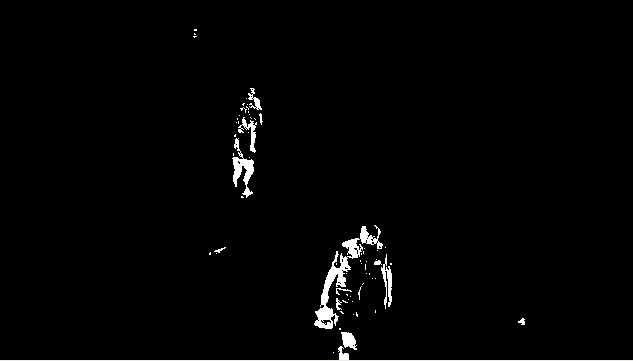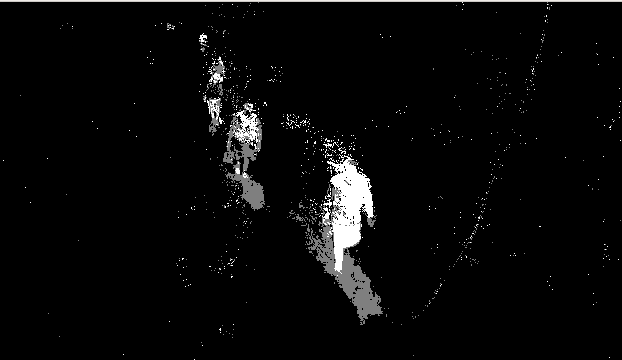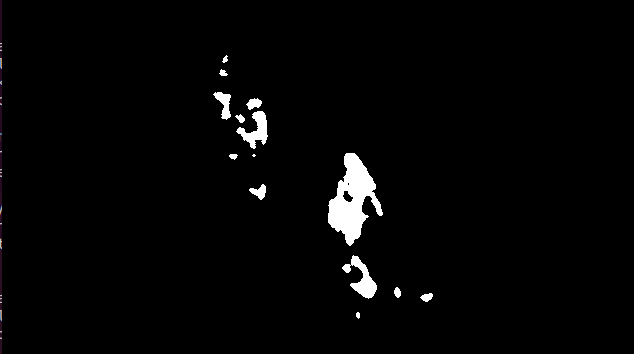# Python OpenCV – Background Subtraction

Background Subtraction is one of the major Image Processing tasks. It is used in various Image Processing applications like Image Segmentation, Object Detection, etc. OpenCV provides us 3 types of Background Subtraction algorithms:-

• BackgroundSubtractorMOG
• BackgroundSubtractorMOG2
• BackgroundSubtractorGMG

Normally, we can perform background Subtraction using matrix subtraction, i.e, just subtracting the static frame from the video. But this has a lot of drawbacks. It is a very less efficient algorithm for Background subtraction because it does not update itself. This problem is being handled by the Background Subtraction algorithms provided by OpenCV.

## Using BackgroundSubtractorMOG

To use BackgroundSubtractorMOG we can use

`cv2.bgsegm.createBackgroundSubtractorMOG()`

Then we can apply it using the “apply” method on each frame of the video. Consider the below example for a better understanding of the topic.

Example:

 `import` `numpy as np ` `import` `cv2 ` ` `  `cap ``=` `cv2.VideoCapture(``'sample.mp4'``) ` ` `  `# initializing subtractor  ` `fgbg ``=` `cv2.bgsegm.createBackgroundSubtractorMOG()  ` ` `  `while``(``1``): ` `    ``ret, frame ``=` `cap.read()        ` ` `  `    ``# applying on each frame ` `    ``fgmask ``=` `fgbg.``apply``(frame)   ` ` `  `    ``cv2.imshow(``'frame'``, fgmask) ` `    ``k ``=` `cv2.waitKey(``30``) & ``0xff` `    ``if` `k ``=``=` `27``: ` `        ``break` ` `  `cap.release() ` `cv2.destroyAllWindows() `

Output :## Using BackgroundSubtractorMOG2

In the previous subtractor worked fairly well but in real-world situations, there is also a presence of shadows. In BackgroundSubtractorMOG2, we can also detect shadows and in the output of the following code, it’s clearly seen. To apply BackgroundSubtractorMOG2, use

`cv2.createBackgroundSubtractorMOG2()`

Example:

 `import` `numpy as np ` `import` `cv2 ` ` `  `cap ``=` `cv2.VideoCapture(``'sample.mp4'``) ` ` `  `# initializing subtractor  ` `fgbg ``=` `cv2.createBackgroundSubtractorMOG2() ` ` `  `while``(``1``): ` `    ``ret, frame ``=` `cap.read() ` `  `  `    ``# applying on each frame ` `    ``fgmask ``=` `fgbg.``apply``(frame) ` ` `  `    ``cv2.imshow(``'frame'``, fgmask)   ` `    ``k ``=` `cv2.waitKey(``30``) & ``0xff` `    ``if` `k ``=``=` `27``: ` `        ``break` ` `  `cap.release() ` `cv2.destroyAllWindows() `

Output :## Using BackgroundSubtractorGMG

This algorithm combines statistical background image estimation and per-pixel Bayesian segmentation. It employs a probabilistic foreground segmentation algorithm that identifies possible foreground objects using Bayesian inference. To use BackgroundSubtractorGMG, use

`cv2.bgsegm.createBackgroundSubtractorGMG()`

Note: We will get a black window during first few frames.

 `import` `numpy as np ` `import` `cv2 ` ` `  `cap ``=` `cv2.VideoCapture(``'sample.mp4'``) ` ` `  `kernel ``=` `cv2.getStructuringElement(cv2.MORPH_ELLIPSE, (``3``, ``3``)) ` ` `  `# initializing subtractor  ` `fgbg ``=` `cv2.bgsegm.createBackgroundSubtractorGMG() ` ` `  `while``(``1``): ` `    ``ret, frame ``=` `cap.read() ` ` `  `    ``# applying on each frame ` `    ``fgmask ``=` `fgbg.``apply``(frame) ` ` `  `    ``fgmask ``=` `cv2.morphologyEx(fgmask, cv2.MORPH_OPEN, kernel)      ` ` `  `    ``cv2.imshow(``'frame'``, fgmask) ` `    ``k ``=` `cv2.waitKey(``30``) & ``0xff` `    ``if` `k ``=``=` `27``: ` `        ``break` ` `  `cap.release() ` `cv2.destroyAllWindows() `

Output :My Personal Notes arrow_drop_upCheck out this Author's contributed articles.

If you like GeeksforGeeks and would like to contribute, you can also write an article using contribute.geeksforgeeks.org or mail your article to contribute@geeksforgeeks.org. See your article appearing on the GeeksforGeeks main page and help other Geeks.

Please Improve this article if you find anything incorrect by clicking on the "Improve Article" button below.

Article Tags :

1

Please write to us at contribute@geeksforgeeks.org to report any issue with the above content.